# RF Tutorial Lesson 17: Simulating a Frequency-Modulated Continuous-Wave (FMCW) Radar System

 Tutorial Project: Simulating a Frequency-Modulated Continuous-Wave (FMCW) Radar System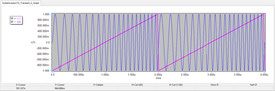Objective: In this project, you will build and test FMCW radar systems with the capability of target range and velocity detection. Concepts/Features: Radar System FMCW Radar Chirp Signal FM Modulation Target Range Target Velocity Beat Frequency Minimum Version Required: All versions

## What You Will Learn

In this tutorial you will use RF.Spice's black-box virtual blocks to model an FMCW radar system. You will explore FMCW radar systems with both sawtooth and triangular chirp modulations and see how the target range and velocity information are extracted from the output beat signals.

## Overview of the FMCW Radar System

In an FMCW radar system, a chirp signal is launched into the free space using a transmit antenna. A chirp signal is an FM-modulated signal of a known stable frequency whose instantaneous frequency varies linearly over a fixed period of time (sweep time) by a modulating signal. The transmitted signal hits at the target and reflects back to a receive antenna. The frequency difference between the received signal and the transmitted signal increases with delay, and the delay is linearly proportional to the range, that is the distance of the target from the radar. The echo from the target is then mixed with the transmitted signal and down-converted to produce a beat signal which is linearly proportional to the target range after demodulation. The figure below shows the transmitted and received ramp signals:The transmitted and received ramp waveforms in an FMCW system with sawtooth chirp modulation.

The delay τ is equal to the round-trip wave travel time and given by:

$\tau = \frac{2R}{c}$

where R is the target range and c is the free-space speed of light. The beat frequency at the output of the receiver is given by:

$f_b = \frac{B}{T_s}\tau$

where B is the total frequency deviation of the chirp signal and Ts is the sweep time (chirp period). The target range is thus found from the following equation:

$R = \frac{cT_s}{2B} f_b$The simplified block diagram of an FMCW radar system.

An FMCW radar system with a sawtooth chirp modulation can only measure the target's range but not its velocity if the target is moving. For that purpose, you need a triangular wave chirp modulation as shown in the figure below: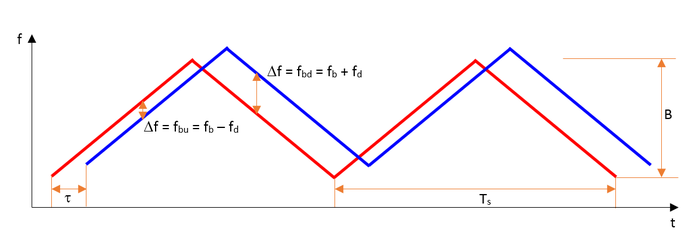The transmitted and received ramp waveforms in an FMCW system with triangular chirp modulation.

A moving target causes addition frequency shifting of the echo signal due to the Doppler effect, which can be expressed by the following relationship:

$f_{Rx} = f_{Tx} \left( \frac{1+v/{c}}{1-v/c} \right)$

where fTx and fRx are the frequency of the transmitted and received signals respectively, and vr is the relative velocity of the target with respect to the radar system. The frequency shift due to the target's velocity is then given by:

$f_d = f_{Rx} - f_{Tx} = 2 f_{Tx}\frac{v_r}{c-v_r} \approx \frac{2v_r}{\lambda_0}$

where and λ0 = c/f is the free=space wavelength, and it was assumed that vr << c. For example, at an operating frequency of f0 = 10GHz, λ0 = 30mm. A moving car target driving at a speed of 20m/s (or 72km/hr) towards the radar generates a frequency shift of fd = 1.33kHz. When driving away from the radar, the same car produces a Doppler shift of -1.33kHz.

The Doppler frequency shift fd caused by a moving target is superposed with the frequency shift of the echo signal due to reflection from a stationary target. When the target is approaching the radar, fd is positive and it slightly lifts up the reflected ramp signal. When the target is moving away from the radar, fd is negative and it slightly lowers down the reflected ramp signal. This leads to generation of two different frequency beat signals during the up-ramp and down-ramp sweeps:

$f_{bu} = f_b - f_d \\ f_{bd} = f_b + f_d$

where fbu and fbd are the beat frequencies during the up-ramp and down-ramp sweeps, respectively. The target range and velocity are then calculated from the following equations:

$R = \frac{cT_s}{4B} \left( f_{bd} + f_{bu} \right)$

$v_r = \frac{\lambda_0}{4} \left( f_{bd} - f_{bu} \right)$

In the above equations, the sweep time Ts is half the period of the ramp's triangular wave function.

## Exploring FM Modulation

The following is a list of parts needed for this part of the tutorial lesson:

Part Name Part Type Part Value
V1 Voltage Source Waveform TBD
X1 FM Modulator Block Defaults
R1 Resistor 100

RF.Spice's FM modulator takes an arbitrary input signal and generates a frequency-modulated sinusoidal signal as its output. Place and connect the parts as shown in the figure below.

In this part of the lesson, you will use different waveforms as the input signal to the FM modulator. You can access the FM Modulator Block from Menu > Parts > Modulation Blocks > FM Modulator Block. The property dialog of this block is shown in the figure below. Set the Carrier Frequency to 10MHz and the Maximum Frequency Deviation to 5MHz.The property dialog of the FM Modulator Block.

You will use the basic voltage source V1 to generate a sinusoidal waveform. Then you will replace it with a Pulse Generator, a Ramp Generator and a Triangular Wave Generator, all of which can be accessed from Menu > Parts > Waveform Generator Blocks > Basic Waveforms. Define each source one by one according to the waveform tables below:

 Offset Voltage 0 1V 0.5MHz 0 0

 Initial Voltage -1 1 0 1n 1n 1u 2u

 Ramp Period 0 -1V 1V 0 (Up-Ramp)

 fo 0.5MHz 0.5 1V

For each waveform, run a Transient Test of your modulator circuit with the following parameters:

 Start Time 0 4u 1n 1n v(1), v(2)

The results are shown in the figures below for all the four different waveforms. Using the graph window's Delta Line Mode, you can measure the period of the FM-modulated waveforms at different time instants. You will find that when the input signal is zero, the period is 100ns (corresponding to 10MHz). When the input signal reaches +1V or -1V, the modulated output frequency increases to 15MHz or decreases to 5MHz, respectively.The graph of the input and output waveforms of the FM modulator circuit with a sinusoidal input.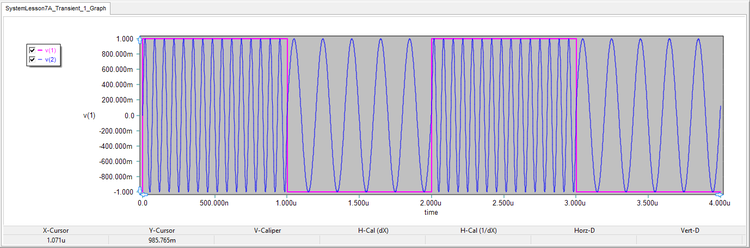The graph of the input and output waveforms of the FM modulator circuit with a pulse (square wave) input.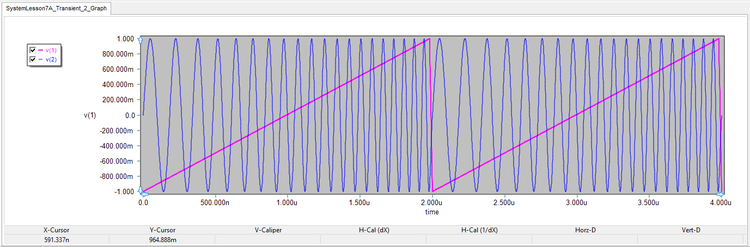The graph of the input and output waveforms of the FM modulator circuit with a ramp (sawtooth wave) input.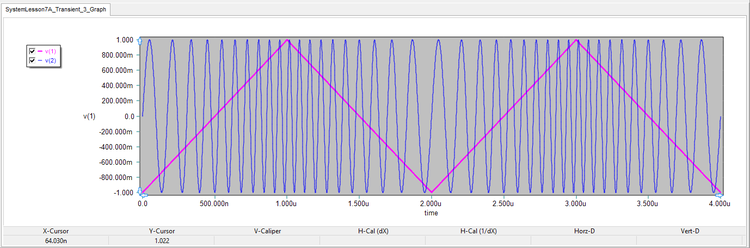The graph of the input and output waveforms of the FM modulator circuit with a triangular wave input.

## Modeling an FMCW System with Sawtooth Modulation

The following is a list of parts needed for this part of the tutorial lesson:

Part Name Part Type Part Value
X1 Ramp Generator Defaults, Chirp Period = 100u, freq_low = 1GHz, freq_high = 1.01GHz
X2 Ideal Signal Splitter Defaults (k = 0.5)
X3 Radar Echo Block Defaults, range = 1500m, fo = 1GHz
X4 Generic Lowpass Filter Block Defaults, Cutoff = 2Meg
E1 Voltage-Controlled Voltage Source Defaults, gain = 1, controlled by v(4,0)
PA Gain Block Defaults, gain = 5e+3
LNA Gain Block (must be made unique) Defaults, gain = 5e+3
AMP Gain Block (must be made unique) Defaults, gain = 50
MIX Multiplier Block Defaults
Rant Resistor 50
RL Resistor 50

In this part of the tutorial lesson, you will build and test an FMCW system with a sawtooth chirp modulation. RF.Spice A/D provides three types of chirp generator devices. All three are based on sawtooth modulation but each provides a different output waveform. They are sinusoidal chirp generator, triangular wave chirp generator and square wave chirp generator. For this project, you will use the first type, which can be access from Menu > Parts > Waveform Generation Blocks > Chirp Generators > Sine Wave Chirp Generator. Set the Chirp Period to 100μs (i.e. a 10kHz chirp), and set the values of the two parameters freq_low and freq_high to 1GHz and 1.01GHz, respectively. Therefore, B = 1.01GHz - 1GHz = 10MHz.The property dialog of the Sine Wave Chirp Generator.

Place and connect all the parts as shown in the figure below. The signal of the chirp generator is split into two equal parts using an Ideal Splitter Block, one of which is amplified by the power amplifier Gain Block PA with a gain of 5e+3 and then goes to a 50Ω matched antenna load called RL. The received signal at the receiver antenna is modeled here using a voltage-controlled voltage source (VCVS) E1 and a Radar Echo Block X3. This block simulates the effect of signal reflection from a target. It cause a delay of its input signal (transmitted signal) as well as its attenuation. The figure below shows the property dialog of the Radar Echo Block. Set the target range equal to 1500m, the frequency fo to 1GHz and keep the default value of σ = 1m2 for the targets radar cross section (RCS).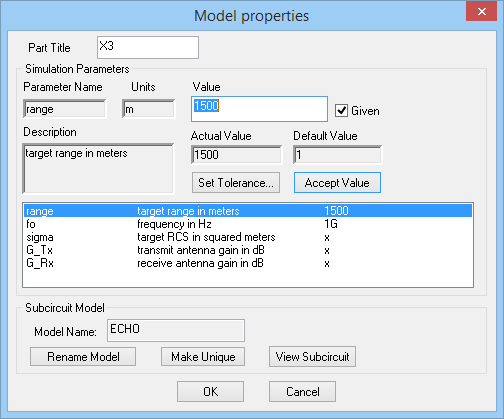The property dialog of the Radar Echo Block.

The controlled source E1 is dependent on the voltage at Node 4, i.e. the antenna load, and creates an exact replica of it that is fed into the echo block. Node 6 indeed represents the receive antenna, or the receiver's entry point. The received signal is amplified by the low-noise amplifier Gain Block LNA with a gain of 5e+3. This signal is then mixed with the other half of the split chirp signal using the Multiplier Block MIX. The down-converted signal is then amplified once more by the Gain Block AMP with a gain of 50 and is passed through a generic lowpass filter block with a cutoff frequency of 2MHz.The schematic of the FMCW radar transmitter-receiver circuit using sawtooth chirp modulation.

Run a Transient Test of your modulator circuit with the following parameters:

 Start Time 0 20u 50p 50p v(1), v(7), v(9), v(10)

Some of the results are shown in the figure below. v(1) is the transmitted chirp signal, and v(7) is the amplified received signal. The blue signal v(10) is the output beat signal, which has a measured period of 1.009μs. Therefore, fb = 1MHz.Radar system simulations usually involve time scales of different orders. They typically require a large transient time interval with a very fine time step ceiling.The graph of the transmitted signal (light blue), received signal (yellow), and the output beat signal (dark blue).

The figure below shows the input and output signals of the lowpass filter, i.e. the mixer's down-converted output signal and the filtered output beat signal: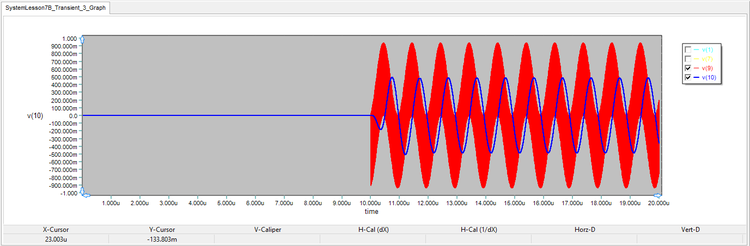The graph of the input and output signals of the lowpass filter.

In this simulation, the stop time was 20μs to cover both the transmitted and received signals adequately. On the other hand, the periods of the signals were on the order of 1ns. Therefore, a step ceiling of 50ps was chosen. From the above figure, you can see that the received signal starts at t = τ = 10μs. You can zoom in the graph to see the details of the transmitted and received signals. The figure below shows the results with a scale time axis limited to the interval [19.99μs, 20μs]:The zoomed-in graph of the transmitted and received signals and the mixer output in the interval [19.99μs, 20μs].

Using the equations given at the beginning of this tutorial lesson, you will find the range of your target to be 1.5km, you had set in the radar echo block.

$R = \frac{cT_s}{2B} f_b = \frac{(3\times 10^8)(100\times 10^{-6})}{2(10\times 10^6)} \left( 10^6 \right) = 1500\text{m}$

## Modeling an FMCW System with Triangular Chirp Modulation

The following is a list of parts needed for this part of the tutorial lesson:

Part Name Part Type Part Value
V1 Triangular Wave Generator Defaults, fo= 20KHz
X1 FM Modulator Block Defaults, fc = 1GHz, f_del = 10MHz
X2 FM Modulator Block Defaults, fc = 1.001GHz, f_del = 10MHz
X3 Ideal Signal Splitter Defaults (k = 0.5)
X4 Radar Echo Block Defaults, range = 1500m, fo = 1GHz
PA Gain Block Defaults, gain = 5e3
A1 Gain Block (copy of PA) Defaults, gain = 5e3
LNA Gain Block (must be made unique) Defaults, gain = 5e3
AMP Gain Block (must be made unique) Defaults, gain = 100
MIX Multiplier Block Defaults
X5 Generic Lowpass Filter Block Defaults, Cutoff = 12Meg
Rant Resistor 50
RL Resistor 50

RF.Spice A/D doesn't offer a sine wave chirp generator with triangular modulation. But you can build one using an FM modulator block just as you did in the first part of this tutorial lesson. You will do this by feeding a triangular wave generator V1 with a frequency of 20kHz (and a period of 50μs) as the modulating signal to the FM Modulator block X1 with a carrier frequency fc = 1GHz and a maximum frequency deviation of 10MHz. As the input triangular wave oscillates between -1V and +1V, the output frequency of the FM modulator will oscillate between 990MHz and 1010MHz. Therefore, B = 20MHz and Ts = 25μs.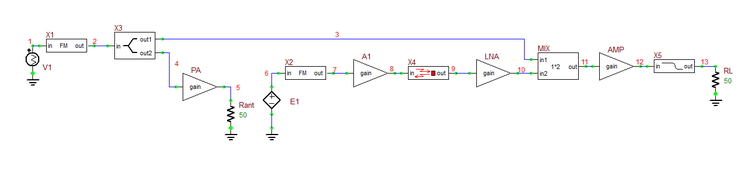The schematic of the FMCW radar transmitter-receiver circuit using triangular chirp modulation.

Place and connect all the parts as shown in the above figure. The circuit of this part is similar to the circuit of the previous part, except for the fact that you need to introduce a Doppler frequency shift in the echo signal. To model this additionally frequency-shifted echo signal, you introduce a second FM modulator X2 which is fed by the same triangular waveform V1 but with a slightly shifted carrier frequency of 1.0001GHz. For the purpose of this tutorial and to demonstrate a practical simulation, we assume that the Doppler frequency shift is fd = 100kHz. This translates to a target velocity of vr = fdλ0/2 = 15km/s, which is extremely high. But remember that typical vehicular speeds would only produce a Doppler shift of few hundreds of Hertz at 1GHz. The VCVS source E1 creates an exact replica of V1 at the input of FM modulator X2 that is amplified by the Gain Block A1, which is a copy of the gain block PA placed before the transmit antenna load Rant. The output of A1 then passes through a Radar Echo Block of the same parameters as the previous part and with a target range of 1500m. In this way, the signal at Node 9 at the output of the radar echo block gives a good representation of the echo signal received at the aperture of the receive antenna.

Run a Transient Test of your modulator circuit with the following parameters:

 Start Time 0 60u 100p 100p v(1), v(13)

The results are shown in the figure below. v(1) is the transmitted triangular ramp signal, and v(13) is the two-frequency output beat signal.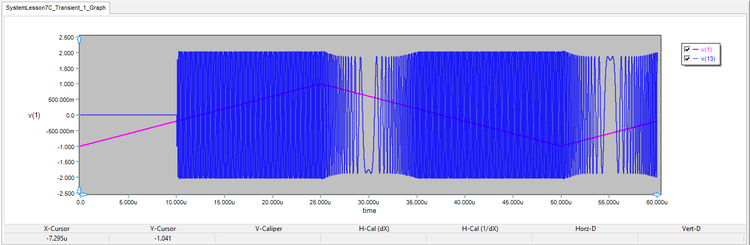The graph of the input triangular ramp signal and the output beat signal of the FMCW radar transmitter-receiver circuit using triangular chirp modulation.

In order to analyze the above results, zoom in the time axis and limit its scale to two intervals: [22μs, 23μs] during the up-ramp and [42μs, 43μs] during the down-ramp. The zoomed-in graphs are shown below.The zoomed-in graph of output beat signal v(13) during the time interval [22μs, 23μs].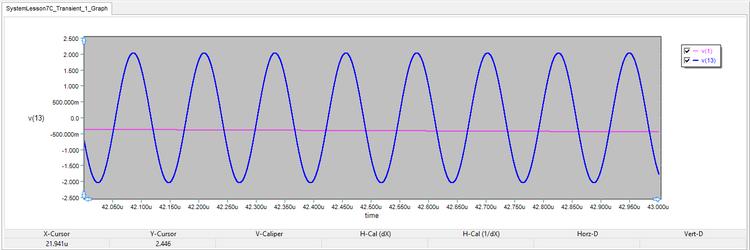The zoomed-in graph of output beat signal v(13) during the time interval [42μs, 43μs].

Read the periods of the output beat signal at the middle of the two zoomed-in time intervals:

Time Instant Ramp Direction Output Period Output Beat Frequency
t = 22.5μs up-ramp 126.5ns fbu = 7.905MHz
t = 42.5μs downp-ramp 123.4ns fbd = 8.103MHz

From the two beat frequencies during the up-ramp and down-ramp, you can calculate the target range and its velocity:

$R = \frac{cT_s}{4B} \left( f_{bd} + f_{bu} \right) = \frac{(3\times 10^8)(25\times 10^{-6})}{4(20\times 10^6)} (8.103 + 7.905)\times 10^6 = 1500.75\text{m}$

$v_r = \frac{\lambda_0}{4} \left( f_{bd} - f_{bu} \right) = \frac{0.3}{4}(8.103 - 7.905)\times 10^6 = 14850\text{m/s}$Back to RF.Spice A/D Tutorial Gateway